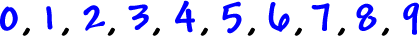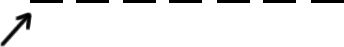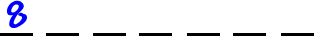Now, we can start to do some cool counting!

Let's figure out how many phone numbers there are in each area code:

Phone numbers have 7 digits...What we'll do is pick the first digit, then the second digit, then the
third, and so on.  (Just like dialing!)  This will allow us to use the
counting principle!

OK, so the possible digits are:Ten possibilities!

Pick the first digit:Typically, I'd say that there would be 10 choices here...  But, we can't start a phone number with a 0 or a 1...  So, there are 8 possibilities here:Now, we pick the second digit -- no restrictions here...10 possibilities

and 10 for each digit after that:Since we picked slot 1, then slot 2, and so on, we get to use the counting principle:So, there are 8,000,000 possible phone numbers.